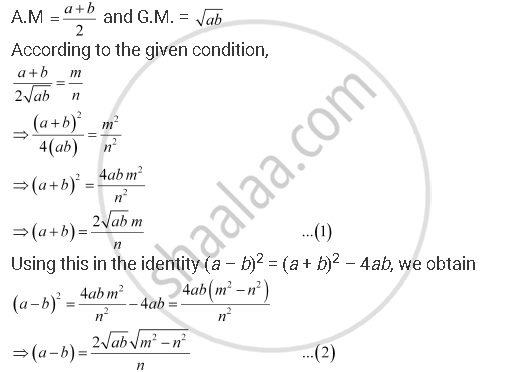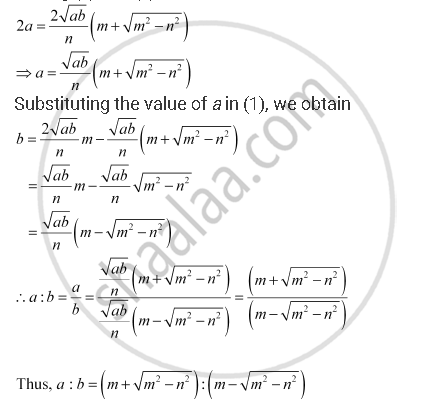CBSE (Arts) Class 11CBSE
Share

# The Ratio of the A.M and G.M. of Two Positive Numbers A And B, Is M: N. Show that A:B = (M + Sqrt(N^2 - N^2)):(M - Sqrt(M^2 - N^2)). - CBSE (Arts) Class 11 - Mathematics

ConceptRelationship Between A.M. and G.M.

#### Question

The ratio of the A.M and G.M. of two positive numbers a and b, is m: n. Show that a:b = (m + sqrt(n^2 - n^2)):(m - sqrt(m^2 - n^2)).

#### Solution

Let the two numbers be a and b.Adding (1) and (2), we obtainIs there an error in this question or solution?

#### APPEARS IN

NCERT Solution for Mathematics Textbook for Class 11 (2018 to Current)
Chapter 9: Sequences and Series
Q: 19 | Page no. 200
Solution The Ratio of the A.M and G.M. of Two Positive Numbers A And B, Is M: N. Show that A:B = (M + Sqrt(N^2 - N^2)):(M - Sqrt(M^2 - N^2)). Concept: Relationship Between A.M. and G.M..
S